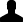Mathematical tools for understanding infectious diseases dynamics [electronic resource] /
Odo Diekmann, Hans Heesterbeek, and Tom Britton.
imprint
Princeton : Princeton University Press, c2013.
description
xiv, 502 p. ; 26 cm.
ISBN
9780691155395 (hardback)
format(s)
Book
HoldingsSingle User
Web
Subjects
More Details
author
imprint
Princeton : Princeton University Press, c2013.
isbn
9780691155395 (hardback)
restrictions
Licensed for access by U. of T. users.
catalogue key
8843333

Includes bibliographical references (p. -496) and index.
A Look Inside
Author Affiliation
Odo Diekmann is professor of applied analysis at Utrecht University. Hans Heesterbeek is professor of theoretical epidemiology at Utrecht University. Tom Britton is professor of mathematical statistics at Stockholm University.
Excerpts
Flap Copy
"This landmark volume describes for readers how one should view the theoretical side of mathematical epidemiology as a whole. A particularly important need is for a book that integrates deterministic and stochastic epidemiological models, and this is the first one that does this. I know of no better overview of the subject. It belongs on the shelf of everyone working in mathematical epidemiology."-- Fred Brauer, University of British Columbia
Flap Copy
"This landmark volume describes for readers how one should view the theoretical side of mathematical epidemiology as a whole. A particularly important need is for a book that integrates deterministic and stochastic epidemiological models, and this is the first one that does this. I know of no better overview of the subject. It belongs on the shelf of everyone working in mathematical epidemiology."--Fred Brauer, University of British Columbia
Summaries
Main Description
Mathematical modeling is critical to our understanding of how infectious diseases spread at the individual and population levels. This book gives readers the necessary skills to correctly formulate and analyze mathematical models in infectious disease epidemiology, and is the first treatment of the subject to integrate deterministic and stochastic models and methods. Mathematical Tools for Understanding Infectious Disease Dynamics fully explains how to translate biological assumptions into mathematics to construct useful and consistent models, and how to use the biological interpretation and mathematical reasoning to analyze these models. It shows how to relate models to data through statistical inference, and how to gain important insights into infectious disease dynamics by translating mathematical results back to biology. This comprehensive and accessible book also features numerous detailed exercises throughout; full elaborations to all exercises are provided. Covers the latest research in mathematical modeling of infectious disease epidemiology Integrates deterministic and stochastic approaches Teaches skills in model construction, analysis, inference, and interpretation Features numerous exercises and their detailed elaborations Motivated by real-world applications throughout
Library of Congress Summary
"Mathematical modeling is critical to our understanding of how infectious diseases spread at the individual and population levels. This book gives readers the necessary skills to correctly formulate and analyze mathematical models in infectious disease epidemiology, and is the first treatment of the subject to integrate deterministic and stochastic models and methods. Mathematical Tools for Understanding Infectious Disease Dynamics fully explains how to translate biological assumptions into mathematics to construct useful and consistent models, and how to use the biological interpretation and mathematical reasoning to analyze these models. It shows how to relate models to data through statistical inference, and how to gain important insights into infectious disease dynamics by translating mathematical results back to biology. This comprehensive and accessible book also features numerous detailed exercises throughout; full elaborations to all exercises are provided. Covers the latest research in mathematical modeling of infectious disease epidemiology Integrates deterministic and stochastic approaches Teaches skills in model construction, analysis, inference, and interpretation Features numerous exercises and their detailed elaborations Motivated by real-world applications throughout "--
Bowker Data Service Summary
Mathematical modeling is critical to our understanding of how infectious diseases spread at the individual & population levels. This book gives readers the necessary skills to correctly forumate & analyze mathematical models in infectious disease epidemiology, & is the first treatment of the subject to integrate deterministic & stochastic models & methods.